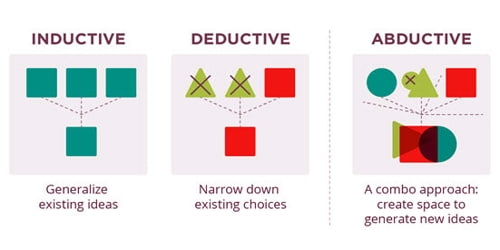Abductive reasoning is to abduce (or take away) a logical assumption, explanation, inference, conclusion, hypothesis, or best guess from an observation or set of observations. Because the conclusion is merely a best guess, the conclusion that is drawn may or may not be true. It is also called abduction, abductive inference, or retroduction, which is a form of logical inference which starts with an observation or set of observations then seeks to find the simplest and most likely explanation.

In the philosophical literature, the term “abduction” is used in two related but different senses. In both senses, the term refers to some form of explanatory reasoning.

Most philosophers agree that abduction (in the sense of Inference to the Best Explanation) is a type of inference that is frequently employed, in some form or other, both in every day and in scientific reasoning.

Abductive reasoning comes in various guises. It can be seen as a way of generating explanations of phenomena meeting certain conditions. One handy way of thinking of it is as “inference to the best explanation”. The exact nature of abduction and the principles for demarcating good and bad abduction is still somewhat of a mystery.

The term “abduction” also sometimes only refers to the generation of hypotheses that explain observations or conclusions, but the former definition is more common both in philosophy and computing.

Aristotle discussed abductive reasoning (apagoge, Greek) in his Prior Analytics. Charles Peirce formulated abduction as a method of scientific research and introduced it into modern logic. The concept of abduction is applied beyond logic to the social sciences and the development of artificial intelligence.

In the 1990s, as computing power grew, the fields of law, computer science, and artificial intelligence research spurred renewed interest in the subject of abduction. Diagnostic expert systems frequently employ abduction.There are three kinds of logical reasoning in logic: Deduction, induction, and abduction.

• Deduction means determining the conclusion. It is using the rule and its precondition to make a conclusion. Example: “When it rains, the grass gets wet. It Rains. Thus, the grass is wet.” Mathematicians are commonly associated with this style of reasoning. A deductive statement is based on accepted truths. For example, all bachelors are unmarried men. It is true by definition and is independent of sense experience.
• Induction means determining the rule. It is learning the rule after numerous examples of the conclusion following the precondition. Example: “The grass has been wet every time it has rained. Thus, when it rains, the grass gets wet.” Scientists are commonly associated with this style of reasoning.
• Abduction means determining the precondition. It is using the conclusion and the rule to assume that the precondition could explain the conclusion. Example: “When it rains, the grass gets wet. The grass is wet, it must have rained.” Diagnosticians and detectives are commonly associated with this style of reasoning.

In logic, an explanation is done from a logical theory T representing a domain and a set of observations O. Abduction is the process of deriving a set of explanations of O according to T and picking out one of those explanations. For E to be an explanation of O according to T, it should satisfy two conditions:

O follows from E and T;

E is consistent with T.

In formal logic, O and E are assumed to be sets of literals. The two conditions for E being an explanation of O according to theory T are formalized as:

T U E |=O

T U E is consistent.

Among the possible explanations E satisfying these two conditions, some other condition of minimality is usually imposed to avoid irrelevant facts (not contributing to the entailment of O) being included in the explanations. Abduction is then the process that picks out some member of E. Criteria for picking out a member representing “the best” explanation includes the simplicity, the prior probability, or the explanatory power of the explanation.

Applications in artificial intelligence include fault diagnosis, belief revision, and automated planning. The most direct application of abduction is that of automatically detecting faults in systems: Given a theory relating faults with their effects and a set of observed effects, abduction can be used to derive sets of faults that are likely to be the cause of the problem.

Abduction can also be used to model automated planning. Given a logical theory relating action occurrences with their effects (for example, a formula of the event calculus), the problem of finding a plan for reaching a state can be modeled as the problem of abducting a set of literals implying that the final state is the goal state.

Information Source: